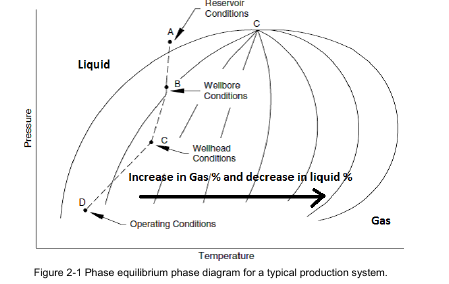# Phase Equilibrium

Equilibrium is a theoretical condition that describes an operating system that has reached a “steady-state”
condition whereby the vapor is condensing to a liquid at exactly the same rate at which liquid is boiling to
vapor. Simply stated, phase equilibrium is a condition where the liquids and vapors have reached certain
pressure and temperature conditions at which they can separate. In most production systems, true
equilibrium is never actually reached; however, vapors and liquids move through the system slow enough
that a “pseudo” or “quasi” equilibrium is assumed. This assumption simplifies process calculations.

Figure 2-1 illustrates several operating points on a generic phase equilibrium diagram. Point A represents
the operating pressure and temperature in the petroleum reservoir. Point B represents the flowing
conditions at the bottom of the production tubing of a well. Point C represents the flowing conditions at the
wellhead. Typically, these conditions are called flowing tubing pressure (FTP) and flowing tubing
temperature (FTT).

Point D represents the surface conditions at the inlet of the first separator.